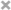## 引言

matplotlib大概是被使用最多的二维绘图Python包。它不仅提供一个非常快捷的用python可视化数据的方法，而且提供了出版质量的多种格式图像。我们将要探索matplotlib包含最常见情况的交互模式。

### Ipython 和 pylab模式

IPython是一个增强的Python交互shell，它拥有很多有趣的特性包括被命名的输入与输出，可使用shell命令，增强的调试和许多其它特性。当我们在命令参数中用-pylab(自从IPython0.12版变成--pylab),它容许交互的matplotlib会话有像Matlab/Mathematica样的功能。

### pylab

pylab提供了一个针对matplotlib面向对象绘图库的程序界面。它模仿Matlab(TM)开发。因此，pylab大部分的绘图命令和参数和Matlab(TM)相似。重要的命令被交互示例解释。

## 简单绘图

from pylab import *

X = np.linspace(-np.pi, np.pi, 256,endpoint=True)
C,S = np.cos(X), np.sin(X)


X现在是一个numpy数组，包含从-π到+π(包含π)等差分布的256个值。C是正弦(256个值)，S是余弦(256个值)。

[[email protected] ~]$ipython2 --pylab Python 2.7.3 (default, Apr 24 2012, 00:00:54) Type "copyright", "credits" or "license" for more information. IPython 0.13 -- An enhanced Interactive Python. ? -> Introduction and overview of IPython's features. %quickref -> Quick reference. help -> Python's own help system. object? -> Details about 'object', use 'object??' for extra details. Welcome to pylab, a matplotlib-based Python environment [backend: Qt4Agg]. For more information, type 'help(pylab)'.  或者你可以下载每个示例然后使用普通的的python运行它： $ python exercice_1.py


### 使用默认matplotlib有一套允许定制各种属性的默认设置。你可以几乎控制matplotlib中的每一个默认属性：图像大小，每英寸点数，线宽，色彩和样式，子图(axes)，坐标轴和网格属性，文字和字体属性，等等。虽然matplotlib的默认设置在大多数情况下相当好，你却可能想要在一些特别的情形下更改一些属性。

from pylab import *

X = np.linspace(-np.pi, np.pi, 256,endpoint=True)
C,S = np.cos(X), np.sin(X)

plot(X,C)
plot(X,S)

show()


### 示例默认# Import everything from matplotlib (numpy is accessible via 'np' alias)
from pylab import *

# Create a new figure of size 8x6 points, using 80 dots per inch
figure(figsize=(8,6), dpi=80)

# Create a new subplot from a grid of 1x1
subplot(1,1,1)

X = np.linspace(-np.pi, np.pi, 256,endpoint=True)
C,S = np.cos(X), np.sin(X)

# Plot cosine using blue color with a continuous line of width 1 (pixels)
plot(X, C, color="blue", linewidth=1.0, linestyle="-")

# Plot sine using green color with a continuous line of width 1 (pixels)
plot(X, S, color="green", linewidth=1.0, linestyle="-")

# Set x limits
xlim(-4.0,4.0)

# Set x ticks
xticks(np.linspace(-4,4,9,endpoint=True))

# Set y limits
ylim(-1.0,1.0)

# Set y ticks
yticks(np.linspace(-1,1,5,endpoint=True))

# Save figure using 72 dots per inch
# savefig("exercice_2.png",dpi=72)

# Show result on screen
show()


### 更改色彩和线宽figure(figsize=(10,6), dpi=80)
plot(X, C, color="blue", linewidth=2.5, linestyle="-")
plot(X, S, color="red",  linewidth=2.5, linestyle="-")


### 设置边界xlim(X.min()*1.1, X.max()*1.1)
ylim(C.min()*1.1, C.max()*1.1)


### 设置刻度xticks( [-np.pi, -np.pi/2, 0, np.pi/2, np.pi])
yticks([-1, 0, +1])


### 设置刻度标签xticks([-np.pi, -np.pi/2, 0, np.pi/2, np.pi],
[r'$-\pi$', r'$-\pi/2$', r'$0$', r'$+\pi/2$', r'$+\pi$'])

yticks([-1, 0, +1],
[r'$-1$', r'$0$', r'$+1$'])


### 移动轴线(spine)ax = gca()
ax.spines['right'].set_color('none')
ax.spines['top'].set_color('none')
ax.xaxis.set_ticks_position('bottom')
ax.spines['bottom'].set_position(('data',0))
ax.yaxis.set_ticks_position('left')
ax.spines['left'].set_position(('data',0))


### 添加图例plot(X, C, color="blue", linewidth=2.5, linestyle="-", label="cosine")
plot(X, S, color="red",  linewidth=2.5, linestyle="-", label="sine")

legend(loc='upper left')


### 注解某些点t = 2*np.pi/3
plot([t,t],[0,np.cos(t)], color ='blue', linewidth=2.5, linestyle="--")
scatter([t,],[np.cos(t),], 50, color ='blue')

annotate(r'$sin(\frac{2\pi}{3})=\frac{\sqrt{3}}{2}$',
xy=(t, np.sin(t)), xycoords='data',
xytext=(+10, +30), textcoords='offset points', fontsize=16,

plot([t,t],[0,np.sin(t)], color ='red', linewidth=2.5, linestyle="--")
scatter([t,],[np.sin(t),], 50, color ='red')

annotate(r'$cos(\frac{2\pi}{3})=-\frac{1}{2}$',
xy=(t, np.cos(t)), xycoords='data',
xytext=(-90, -50), textcoords='offset points', fontsize=16,


## 魔鬼在于细节for label in ax.get_xticklabels() + ax.get_yticklabels():
label.set_fontsize(16)
label.set_bbox(dict(facecolor='white', edgecolor='None', alpha=0.65 ))


### 图像，子区，子图，刻度(Figures,Subplots,Axes,Ticks)

Note:这一段很绕，我不知道该怎么翻译好。在matplotlib中axes容器处于核心地位。翻译成子图因为在《Python科学计算》中作者这样称呼.subplot翻译成子区

### 图像(Figure)

num 1 图像编号
figsize figure.figsize 图像大小(宽度，高度)(英寸)
dpi figure.dpi 每英寸分辨率
facecolor figure.facecolor 背景色
edgecolor figure.edgecolor 绘图背景边沿色
frameon True 绘制框架与否

### 子区(subplots)### 子图(axes)### 刻度(ticks)

#### 刻度定位器(Tick Locator)

Class Description
NullLocator 没有刻度.IndexLocator 每到一个基数的倍数点放置一个刻度FixedLocator 刻度位置是固定的LinearLocator 决定刻度位置MultipleLocator 在每个基于基数倍数的整数点放置刻度AutoLocator 在不错的位置选择不超过n个间隔LogLocator 以log坐标决定刻度位置## 其它种类绘图

### 常规绘图from pylab import *

n = 256
X = np.linspace(-np.pi,np.pi,n,endpoint=True)
Y = np.sin(2*X)

plot (X, Y+1, color='blue', alpha=1.00)
plot (X, Y-1, color='blue', alpha=1.00)
show()


### 散点图(scatter plots)from pylab import *

n = 1024
X = np.random.normal(0,1,n)
Y = np.random.normal(0,1,n)

scatter(X,Y)
show()


### 条形图(bar plots)from pylab import *

n = 12
X = np.arange(n)
Y1 = (1-X/float(n)) * np.random.uniform(0.5,1.0,n)
Y2 = (1-X/float(n)) * np.random.uniform(0.5,1.0,n)

bar(X, +Y1, facecolor='#9999ff', edgecolor='white')
bar(X, -Y2, facecolor='#ff9999', edgecolor='white')

for x,y in zip(X,Y1):
text(x+0.4, y+0.05, '%.2f' % y, ha='center', va= 'bottom')

ylim(-1.25,+1.25)
show()


### 等高线图(contour plots)from pylab import *

def f(x,y): return (1-x/2+x**5+y**3)*np.exp(-x**2-y**2)

n = 256
x = np.linspace(-3,3,n)
y = np.linspace(-3,3,n)
X,Y = np.meshgrid(x,y)

contourf(X, Y, f(X,Y), 8, alpha=.75, cmap='jet')
C = contour(X, Y, f(X,Y), 8, colors='black', linewidth=.5)
show()


### Imshowfrom pylab import *

def f(x,y): return (1-x/2+x**5+y**3)*np.exp(-x**2-y**2)

n = 10
x = np.linspace(-3,3,4*n)
y = np.linspace(-3,3,3*n)
X,Y = np.meshgrid(x,y)
imshow(f(X,Y)), show()


### 饼图(Pie charts)from pylab import *

n = 20
Z = np.random.uniform(0,1,n)
pie(Z), show()


### 矢量图(quiver plots)from pylab import *

n = 8
X,Y = np.mgrid[0:n,0:n]
quiver(X,Y), show()


### 网格(grids)from pylab import *

axes = gca()
axes.set_xlim(0,4)
axes.set_ylim(0,3)
axes.set_xticklabels([])
axes.set_yticklabels([])

show()


### 多图绘制from pylab import *

subplot(2,2,1)
subplot(2,2,3)
subplot(2,2,4)

show()


### 极轴图from pylab import *

axes([0,0,1,1])

N = 20
theta = np.arange(0.0, 2*np.pi, 2*np.pi/N)
width = np.pi/4*np.random.rand(N)
bars = bar(theta, radii, width=width, bottom=0.0)

bar.set_facecolor( cm.jet(r/10.))
bar.set_alpha(0.5)

show()


### 三维绘图from pylab import *
from mpl_toolkits.mplot3d import Axes3D

fig = figure()
ax = Axes3D(fig)
X = np.arange(-4, 4, 0.25)
Y = np.arange(-4, 4, 0.25)
X, Y = np.meshgrid(X, Y)
R = np.sqrt(X**2 + Y**2)
Z = np.sin(R)

ax.plot_surface(X, Y, Z, rstride=1, cstride=1, cmap='hot')

show()


### 绘制文本## 此教程之外

matplotlib受益于丰富的文档和巨大的用户和开发者社区。这有几个有关的链接：

pyplot教程

artist对象教程

### 代码文档

>>> from pylab import *
>>> help(plot)
Help on function plot in module matplotlib.pyplot:

plot(*args, **kwargs)
Plot lines and/or markers to the
:class:~matplotlib.axes.Axes.  *args* is a variable length
argument, allowing for multiple *x*, *y* pairs with an
optional format string.  For example, each of the following is
legal::

plot(x, y)         # plot x and y using default line style and color
plot(x, y, 'bo')   # plot x and y using blue circle markers
plot(y)            # plot y using x as index array 0..N-1
plot(y, 'r+')      # ditto, but with red plusses

If *x* and/or *y* is 2-dimensional, then the corresponding columns
will be plotted.


## 快速参考